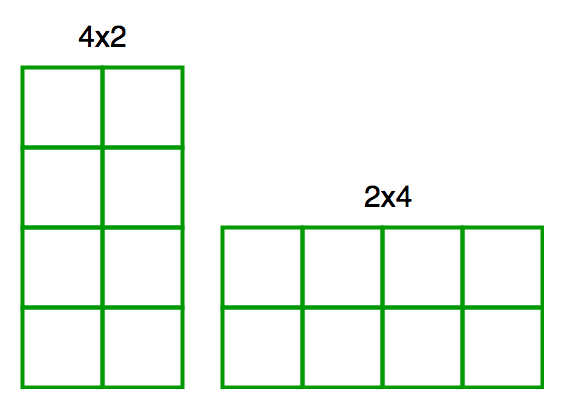# Number of unique rectangles formed using N unit squares

• Difficulty Level : Medium
• Last Updated : 28 Apr, 2021

You are given N unit squares (squares with side length 1 unit), and you are asked to make rectangles using these squares. You have to count the number of rotationally unique rectangles than you can make. What does rotationally unique mean? Well, two rectangles are rotationally unique if one can’t be rotated to become equivalent to the other one.

Example – The 4×2 rectangle can be rotated 90 degrees clockwise to make it the exact same as the 2×4 rectangle and so these are not rotationally unique.

Attention reader! Don’t stop learning now. Get hold of all the important DSA concepts with the DSA Self Paced Course at a student-friendly price and become industry ready.  To complete your preparation from learning a language to DS Algo and many more,  please refer Complete Interview Preparation Course.

In case you wish to attend live classes with experts, please refer DSA Live Classes for Working Professionals and Competitive Programming Live for Students.Examples :

```Input : N = 4
Output : 5
We can make following five rectangles
1 x 1, 1 x 2, 2 x 2, 1 x 3 and 1 x 4

Input : N = 5
Output : 6

Input : 6
Output : 8```

So how do we solve this problem?
Every rectangle is uniquely determined by its length and its height.
A rectangle of length = l and height = h then l * h <= n is considered equivalent to a rectangle with length = h and height = l provided l is not equal to h. If we can have some sort of “ordering” in these pairs then we can avoid counting (l, h) and (h, l) as different rectangles. One way to define such an ordering is:

Assume that length <= height and count for all such pairs such that length*height <= n.
We have, length <= height
or, length*length <= length*height
or, length*length <= n
or, length <= sqrt(n)

## C++

 `// C++ program to count rotationally equivalent``// rectangles with n unit squares``#include``using` `namespace` `std;` `int` `countRect(``int` `n)``{``    ``int` `ans = 0;``    ``for` `(``int` `length = 1; length <= ``sqrt``(n); ++length)``    ``for` `(``int` `height = length; height*length <= n; ++height)``            ``// height >= length is maintained``        ``ans++;``    ``return` `ans;``}` `// Driver code``int` `main() {``    ``int` `n = 5;``    ``printf``(``"%d"``, countRect(n));``    ``return` `0;``}`

## Java

 `// Java program to count rotationally equivalent``// rectangles with n unit squares``class` `GFG {``    ` `    ``static` `int` `countRect(``int` `n)``    ``{``        ``int` `ans = ``0``;``        ` `        ``for` `(``int` `length = ``1``; length <= Math.sqrt(n);``                                           ``++length)``            ``for` `(``int` `height = length; height*length <= n;``                                                ``++height)``                ``// height >= length is maintained``                ``ans++;``            ` `        ``return` `ans;``    ``}``    ` `    ``//driver code``    ``public` `static` `void` `main (String[] args)``    ``{``        ``int` `n = ``5``;``        ` `        ``System.out.print(countRect(n));``    ``}``}` `// This code is contributed by Anant Agarwal.`

## Python3

 `# Python3 program to count rotationally``# equivalent rectangles with n unit squares``import` `math` `def` `countRect(n):` `    ``ans ``=` `0``    ``for` `length ``in` `range``(``1``, ``int``(math.sqrt(n)) ``+` `1``):``        ``height ``=` `length``        ``while``(height ``*` `length <``=` `n):``            ` `            ``# height >= length is maintained``            ``ans ``+``=` `1``            ``height ``+``=` `1``    ``return` `ans` `# Driver code``n ``=` `5``print``(countRect(n))` `# This code is contributed by Anant Agarwal.`

## C#

 `// C# program to count rotationally``// equivalent rectangles with n unit``// squares``using` `System;` `class` `GFG {``    ` `    ``static` `int` `countRect(``int` `n)``    ``{``        ``int` `ans = 0;``        ``for` `(``int` `length = 1;``            ``length <= Math.Sqrt(n); ++length)``            ` `            ``for` `(``int` `height = length;``                     ``height*length <= n; ++height)``                ``ans++;``                ` `        ``return` `ans;``    ``}``    ` `    ``//driver code``    ``public` `static` `void` `Main()``    ``{``        ` `        ``int` `n = 5;``        ` `        ``Console.Write(countRect(n));``    ``}``}` `//This code is contributed by Anant Agarwal.`

## PHP

 `= length is maintained``        ``\$ans``++;``    ``return` `\$ans``;``}` `// Driver code``\$n` `= 5;``echo` `countRect(``\$n``);` `// This code is contributed by @ajit``?>`

## Javascript

 ``

Output :

`6 `

This article is contributed by Hemang Sarkar. If you like GeeksforGeeks and would like to contribute, you can also write an article using write.geeksforgeeks.org or mail your article to contribute@geeksforgeeks.org. See your article appearing on the GeeksforGeeks main page and help other Geeks.